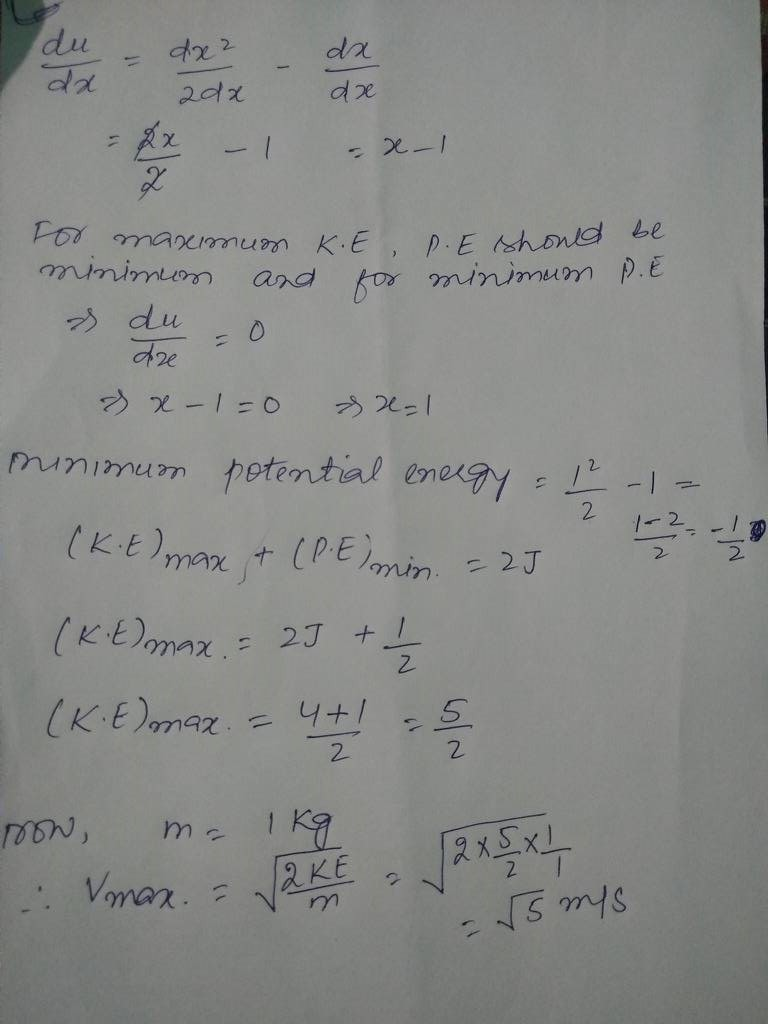×#### Thank you for registering.

One of our academic counsellors will contact you within 1 working day.

Click to Chat

1800-1023-196

+91-120-4616500

CART 0

• 0

MY CART (5)

Use Coupon: CART20 and get 20% off on all online Study Material

ITEM
DETAILS
MRP
DISCOUNT
FINAL PRICE
Total Price: Rs.

There are no items in this cart.
Continue Shopping
```
The potential energy of a particle of mass 1kg moving along x-axis is given by U(x)=[x^2/2-x]J. If total mechanical energy of the particle is 2J find its maximum speed.
The potential energy of a particle of mass 1kg moving along x-axis is given by U(x)=[x^2/2-x]J. If total mechanical energy of the particle is 2J find its maximum speed.

```
4 years ago

```							Ans- 2Speed will be max when kinetic energy is maximum so potential energy will be minimum so differentiating the function put it equal to 0  so we obtain minimum for x=0 now kin potential energy is 0 and kinetic energy will be 2 .mv^2/2 is equal to 2 m  is 1 kg  therefore v=2 .
```
4 years ago
```							Yes I agree when kinetic energy is maximum velocity is maximum and for this potential energy has to be minimum which is taken as 0. but the answer is given as √5m/s.
```
4 years ago
```							total mechanical energy = U+ K.E  to attain maximum speed the obect must have maximum K.E as K.E will be maximum , U has to be minimum (conservation of energy )given - U(x) = x²/2-xfor this polynomial to be minimum x has to be equal to zero [ U`(x) = (2-x)2x +x²/(2-x) =0 then x =0,4 there is minima at x=0]so putting x =0 iñ U(x) we get U=0 therfore total mechanical energy =2 = 0+K.E(max)then K.Emax =2 1/2 mv² =2 then v²=4therefore v=2m/s
```
4 years ago
```							We need to differentiate the equation and we will get value of x. x=1 then substituting x=1 in the given equation will give value of U. Substitute U and total energy=2 in TE = KE + PE. Then we will get v =√5m/s
```
4 years ago
```							What is the denominator of function . I had taken the denominator as x-2 is this correct or not According to this answer coming out is x =0 minimum and x= 4 max for p.e Is this correct or not
```
4 years ago
```							The function is U = [ x²/2 - x ] So differentiate this we get x=1, substituting x=1 in the function we get U = -1/2 then we can find out ke and v
```
4 years ago
```							We know that the object accelerate till force is applied on it and it attains maximum velocity just after the force becomes zero...  SO we know the negative of  potential energy gradient is force..  So differentiate it and put energy gradient equal to 0..  So at X =1 the force Is 0, get the potential energy, subtract it from total energy to get kinetic energy and find its velocity it will be 5^1/2
```
4 years ago
```							U={square of the x divided by the 2 and substracted by x} so differentiate this we get x=1 substituting x=1 in the functio we get U=-1 by 2.
```
3 years ago
```							Potential energy needed to be zero for getting maximum speed..U(x)=[x^2/2 - x] i.e, force will remain constant for it therefore differentiating U(x) wrt d(x) we get x-1for maximum speed d(U(x))/d(x) =0 therefore x=1 T.E = K.E+ P.E2 = 1/2mv^2 + x^2/2 - x=>2 =1/2mv^2 +1/2-1=>2=1/2mv^2 -1/2=>5/2=1/2 v^2.                    (Since m=1)=>v=√5 m/s
```
2 years ago
```							For speed to be maximum potential energy should be minimum. So, differentiate the x^2/2 - x and u will get x-1 on differentiating. Equate it to zero and solve for x. U will get x=1. Now, put x= 1 in the equation U(x) = x^2/2 - 2 to get the value of U which is 1/2. T.E= P.E + K.E. putting P.E = 1/2, K.E= 1/2*m*v^2 where m is given 1kg and finally T.E= 2J in the equation given above we will get v= √5m/s
```
2 years agoYash Chourasiya
256 Points
```							Dear StudentPlease see the solution in the attachment.I hope this answer will help you.Thanks & RegardsYash Chourasiya
```
6 months ago
Think You Can Provide A Better Answer ?

## Other Related Questions on Mechanics

View all Questions »### Course Features

• 101 Video Lectures
• Revision Notes
• Previous Year Papers
• Mind Map
• Study Planner
• NCERT Solutions
• Discussion Forum
• Test paper with Video Solution### Course Features

• 110 Video Lectures
• Revision Notes
• Test paper with Video Solution
• Mind Map
• Study Planner
• NCERT Solutions
• Discussion Forum
• Previous Year Exam Questions